# Maximization and minimization of functions

(diff) ← Older revision | Latest revision (diff) | Newer revision → (diff)

of a finite number of variables

The problem of finding the extrema of a function,. This means

1) finding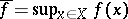or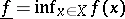;

2) searching maximum or minimum points if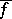or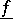are attained on the admissible set (see Maximum and minimum of a function);

3) the construction of maximizing or minimizing sequencessuch that: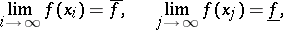iforis not attained on.

The investigation of the extrema of functions of discrete arguments is referred to as discrete programming or integer programming. Below only maximization and minimization for functions of continuous arguments is explained.

The classical (indirect) methods of maximization and minimization apply only to smooth functions. They use necessary conditions for an extremum in order to locate stationary points. Zeros of the derivatives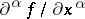,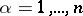, are usually computed in practice by some successive approximation method (see ). On the other hand, each problem of solving a finite set of functional equations of the form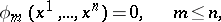can be interpreted as a maximization or minimization problem of some function, for example, of the function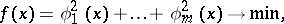and one of the specific methods for maximizing and minimizing can be applied.

Direct methods for maximizing and minimizing functions are based on direct comparison of the values ofat two or more points.

The practical search of extrema uses iterative algorithms of the form: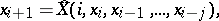whereis the index of the iteration and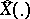is some operator. Here it is usually assumed that

a) the algorithm converges in some sense, most often in the sense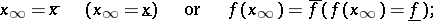b) the iteration procedure is local, that is,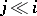(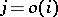as); the algorithm "remembers" the values ofonly for iterations in some neighbourhood of the current position. For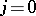a memoryless simple Markov computational process is obtained.

The operatormay be deterministic, in deterministic methods, or may contain stochastic parameters. In computational practice stochastic methods are often combined with deterministic methods, for example, in the coordinate-wise descent method the direction of descent may be determined randomly. The probabilistic characteristics of the stochastic parameters, in turn, may be changed from iteration to iteration (search with adaptation and "self-learning" random search).

Various deterministic methods are widely used in combination, including sequential and parallel computation of an extremum by several methods, composition of algorithms of the form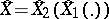, etc. For example, the Levenberg–Marquardt method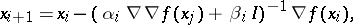which coincides with the gradient method forand with the Newton method for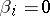.

One-dimensional optimization, that is, maximizing and minimizing a function,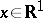, in addition to its interest by itself, is a necessary stage in most applicable methods. Specific one-dimensional methods include, for example, the Fibonacci method; the dichotomy method (division by halves) and the parabola method. Methods for maximizing and minimizing functions in several variables are the gradient method, the method of steepest descent (cf. Steepest descent, method of), the coordinate-wise descent method, the simplex method, the scanning method, the method of conjugate gradients, the method of the heavy sphere (cf. Conjugate gradients, method of; Heavy sphere, method of the), the adjustment method, and others.

The algorithms of most of the methods listed fall into the scheme of the descent (ascent) method: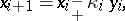where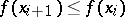or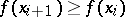for all(the relaxation condition). These are distinguished either by the choice of a descent direction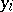or by the method of moving along the descent vector, defined by the step factor.

Cut methods have been developed for functions whose contours are "valleys with steep slopes" (see Minimization methods for functions depending strongly on a few variables). Ordinary (non-cut) methods when applied here give a zig-zag relaxational path, requiring excessive amounts of machine time to calculate the extremum.

The comparative effectiveness of the methods is estimated by many, even contradictory, criteria. E.g., accuracy of the solution, speed of approach to the solution, reliability of the method, preparation time from the problem to the calculation, convergence of the algorithm, etc. The domain of applicability of each of the approved methods is very limited.

For testing the methods a set of standard test functions, characteristic of different function classes, has been collected (see ). Convergence of methods for maximizing and minimizing functions has been extensively studied (see , ). However, convergence is a property which is neither necessary nor sufficient for the effective termination of a calculation.

All methods above lead to a local extremum if the first approximation belongs to the domain of attraction of this extremum. The detection of a global extremum is guaranteed only for convex and related unimodal functions. The theory of finding a global extremum is still (1989) in the initial phase of development (see Multi-extremum problem). Another area of maximizing and minimizing of functions which is developing is optimization of non-smooth functions (see , , ). In particular, the problem of minimizing the maxima of a family of functions generally leads to non-smooth functions (see Maximin, numerical methods). Apparently, all of the commonly-used methods for optimization have an interesting physical, economic or biological meaning. The corresponding research is only just developing (see ) and leads to the creation of new methods (see also Continuous analogues of iteration methods). If the values of the functions being considered are defined statistically with random noise, then one of the methods of stochastic approximation is applied to find extrema. Here one is bordering on the design of experiments.

Experimental methods of maximizing and minimizing functions use the reproduction of various physical processes in the search for extrema. A related area is simulation on analogue computers (see ). In spite of the convenience and cheapness of using the simplest automatic optimizers, they do not guarantee high accuracy of calculation.

Graphical methods are suitable only for obtaining rough estimates and for the construction of a first approximation for iterative methods.

If the admissible set is given by functional conditions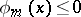(constraints and restrictions, conditional extremum), then methods of mathematical programming are applied in the search of extrema. This problem can be reduced to a sequence of problems on unconstrained extrema by the use of penalty and barrier functions (see Penalty functions, method of).

How to Cite This Entry:
Maximization and minimization of functions. Encyclopedia of Mathematics. URL: http://encyclopediaofmath.org/index.php?title=Maximization_and_minimization_of_functions&oldid=16029
This article was adapted from an original article by Yu.P. IvanilovV.V. Okhrimenko (originator), which appeared in Encyclopedia of Mathematics - ISBN 1402006098. See original article